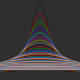# Making Back-to-Back Histograms

A colleage of mine asked me how to do back to back histograms (instead of on top of each other). I feel as though there should be a function like voilin plot from the vioplot package. Voilin plots are good for displaying data, but the violin must have the left and right (or top and bottom) of the violin to be from the same distribution, and therefore are symmetrical. Many times people want to compare two distributions.

Cookbook for R ) shows how to overlay histograms (or densities) on top of each other, so go there if that's what you want. (NB: that is the way I tend to compare distributions, especially more than 2. I provide the code below because some have different preferences.)

### ggplot implementation:

```library(ggplot2)
df = data.frame(x = rnorm(100), x2 = rnorm(100, mean=2))

g = ggplot(df, aes(x)) + geom_histogram( aes(x = x, y = ..density..),
binwidth = diff(range(df\$x))/30, fill="blue") +
geom_histogram( aes(x = x2, y = -..density..), binwidth = diff(range(df\$x))/30, fill= "green")
print(g)
```
```## Warning: Stacking not well defined when ymin != 0
```I simply simulated 2 normal distributions of 100 points and then plotted them. Not the `..density` call in the `aes` for the histograms. This just scales the histogram to a density and not a count. The `-..density..` flips the second histogram around zero so that they are back-to-back. We see that `ggplot` doesn't like stacking when you have negative data, but it's ok for this exmaple and don't overlap.

```print(g + coord_flip())
```
```## Warning: Stacking not well defined when ymin != 0
```Using `coord_flip` plots back-to-back histograms horizontally. This code can easily be extended using `geom_density` and actually a volcano plot version is in the help for `stat_density`.

### Base implementation

Not everyone likes `ggplot2` so I figured I would provide in implementation in base graphics.

```## using base
h1 = hist(df\$x, plot=FALSE)
h2 = hist(df\$x2, plot=FALSE)
h2\$counts = - h2\$counts
hmax = max(h1\$counts)
hmin = min(h2\$counts)
X = c(h1\$breaks, h2\$breaks)
xmax = max(X)
xmin = min(X)
plot(h1, ylim=c(hmin, hmax), col="green", xlim=c(xmin, xmax))
lines(h2, col="blue")
```The code calculates the histograms for each distribution and stores the information. I simply take the negative number of counts to flip the histogram over the x-axis.

### Go forth and prosper

You can adjust the axes to positive numbers, make more implementations with densities/etc, but this is a simple graphic I've seen people use. Hope this helps someone out.

## 5 thoughts on “Making Back-to-Back Histograms”

1.Andrew says:

It is possibly worth noting that histbackback in the outstanding Hmisc library by Frank Harrell has had provided functionality for this for about a decade or more.

http://www.inside-r.org/packages/cran/Hmisc/docs/histbackback

•strictlystat says:

That’s a good point. I hadn’t seen that before. I may do well to just check out Hmisc (which has loads of other stuff I use) before making anything new. That said, I did want a ggplot implementation too. Also, it’s not so easy to add color to histbackback, but it’s not really necessary.

2.Maxwell Joseph says:

Nice post! Split violin plots that are asymmetrical provide another option to compare distributions: http://mbjoseph.github.io/blog/2013/06/24/violin/

•strictlystat says:

Thanks for the reply – I’ve been looking for these but didn’t run into your post. I thought about tweaking vioplot, but didn’t have the time/need. I will use yours going forward – I like it. Thoughts on wrapping it into vioplot and contacting contrib package authors?